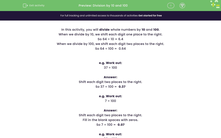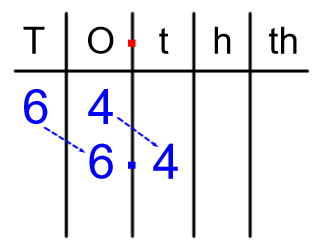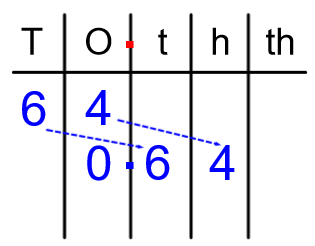# Division by 10 and 100

In this worksheet, students must divide whole numbers by 10 and 100.Key stage:  KS 2

Curriculum topic:   Number: Multiplication and Division

Curriculum subtopic:   Multiply/Divide Using Decimals

Difficulty level:#### Worksheet Overview

In this activity, you will divide whole numbers by 10 and 100.

When we divide by 10, we shift each digit one place to the right.

So 64 ÷ 10 = 6.4When we divide by 100, we shift each digit two places to the right.

So 64 ÷ 100 =  0.64

Notice how we needed to put a place holder in the ones column to show there are 0 ones.e.g. Work out:

37 ÷ 100

Shift each digit two places to the right.

So 37 ÷ 100 =  0.37

e.g. Work out:

7 ÷ 100

Shift each digit two places to the right.

We need to use place holders in the ones and tenths columns to show there are 0 ones and 0 tenths.

So 7 ÷ 100 =  0.07

e.g. Work out:

30 ÷ 100

Shift each digit two places to the right.

Leave off the last zero - we don't need to show that there are no hundredths.

So 30 ÷ 100 =  0.30 = 0.3

### What is EdPlace?

We're your National Curriculum aligned online education content provider helping each child succeed in English, maths and science from year 1 to GCSE. With an EdPlace account you’ll be able to track and measure progress, helping each child achieve their best. We build confidence and attainment by personalising each child’s learning at a level that suits them.

Get started